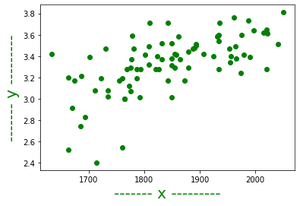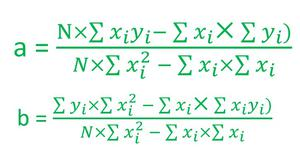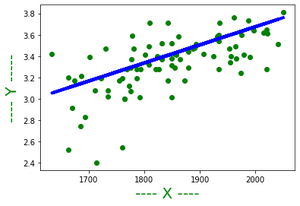# Regression Analysis and the Best Fitting Line using C++

• Last Updated : 28 Jul, 2022

This article discusses the basics of linear regression and its implementation in the C++ programming language. Regression analysis is the common analysis method that is used by data scientists for the prediction of values corresponding to some input data.

The simple regression analysis method is linear regression. Linear regression is a statistical method for modeling relationships between a dependent variable with a given set of independent variables. We are going to implement the same in C++.

The input data set generally comes in a .csv (comma-separated values) file which we will copy from that file and put in a .txt file as an input file for our C++ program. To take input from a file create an input.txt named file in the same directory as the program file and put the data inside that file such that the first line has the value of N i.e the number of entries in that dataset.

Implementation :

## C++

 `// C++ program to implement` `// the above approach` `#include ` `#include ` `#include ` `using` `namespace` `std;` `class` `regression {` `    ``// Dynamic array which is going` `    ``// to contain all (i-th x)` `    ``vector<``float``> x;`   `    ``// Dynamic array which is going` `    ``// to contain all (i-th y)` `    ``vector<``float``> y;`   `    ``// Store the coefficient/slope in` `    ``// the best fitting line` `    ``float` `coeff;`   `    ``// Store the constant term in` `    ``// the best fitting line` `    ``float` `constTerm;`   `    ``// Contains sum of product of` `    ``// all (i-th x) and (i-th y)` `    ``float` `sum_xy;`   `    ``// Contains sum of all (i-th x)` `    ``float` `sum_x;`   `    ``// Contains sum of all (i-th y)` `    ``float` `sum_y;`   `    ``// Contains sum of square of` `    ``// all (i-th x)` `    ``float` `sum_x_square;`   `    ``// Contains sum of square of` `    ``// all (i-th y)` `    ``float` `sum_y_square;`   `public``:` `    ``// Constructor to provide the default` `    ``// values to all the terms in the` `    ``// object of class regression` `    ``regression()` `    ``{` `        ``coeff = 0;` `        ``constTerm = 0;` `        ``sum_y = 0;` `        ``sum_y_square = 0;` `        ``sum_x_square = 0;` `        ``sum_x = 0;` `        ``sum_xy = 0;` `    ``}`   `    ``// Function that calculate the coefficient/` `    ``// slope of the best fitting line` `    ``void` `calculateCoefficient()` `    ``{` `        ``float` `N = x.size();` `        ``float` `numerator` `            ``= (N * sum_xy - sum_x * sum_y);` `        ``float` `denominator` `            ``= (N * sum_x_square - sum_x * sum_x);` `        ``coeff = numerator / denominator;` `    ``}`   `    ``// Member function that will calculate` `    ``// the constant term of the best` `    ``// fitting line` `    ``void` `calculateConstantTerm()` `    ``{` `        ``float` `N = x.size();` `        ``float` `numerator` `            ``= (sum_y * sum_x_square - sum_x * sum_xy);` `        ``float` `denominator` `            ``= (N * sum_x_square - sum_x * sum_x);` `        ``constTerm = numerator / denominator;` `    ``}`   `    ``// Function that return the number` `    ``// of entries (xi, yi) in the data set` `    ``int` `sizeOfData()` `    ``{` `        ``return` `x.size();` `    ``}`   `    ``// Function that return the coefficient/` `    ``// slope of the best fitting line` `    ``float` `coefficient()` `    ``{` `        ``if` `(coeff == 0)` `            ``calculateCoefficient();` `        ``return` `coeff;` `    ``}`   `    ``// Function that return the constant` `    ``// term of the best fitting line` `    ``float` `constant()` `    ``{` `        ``if` `(constTerm == 0)` `            ``calculateConstantTerm();` `        ``return` `constTerm;` `    ``}`   `    ``// Function that print the best` `    ``// fitting line` `    ``void` `PrintBestFittingLine()` `    ``{` `        ``if` `(coeff == 0 && constTerm == 0) {` `            ``calculateCoefficient();` `            ``calculateConstantTerm();` `        ``}` `        ``cout << ``"The best fitting line is y = "` `             ``<< coeff << ``"x + "` `<< constTerm << endl;` `    ``}`   `    ``// Function to take input from the dataset` `    ``void` `takeInput(``int` `n)` `    ``{` `        ``for` `(``int` `i = 0; i < n; i++) {` `            ``// In a csv file all the values of` `            ``// xi and yi are separated by commas` `            ``char` `comma;` `            ``float` `xi;` `            ``float` `yi;` `            ``cin >> xi >> comma >> yi;` `            ``sum_xy += xi * yi;` `            ``sum_x += xi;` `            ``sum_y += yi;` `            ``sum_x_square += xi * xi;` `            ``sum_y_square += yi * yi;` `            ``x.push_back(xi);` `            ``y.push_back(yi);` `        ``}` `    ``}`   `    ``// Function to show the data set` `    ``showData()` `    ``{` `        ``for` `(``int` `i = 0; i < 62; i++) {` `            ``printf``(``"_"``);` `        ``}` `        ``printf``(``"\n\n"``);` `        ``printf``(``"|%15s%5s %15s%5s%20s\n"``,` `               ``"X"``, ``""``, ``"Y"``, ``""``, ``"|"``);`   `        ``for` `(``int` `i = 0; i < x.size(); i++) {` `            ``printf``(``"|%20f %20f%20s\n"``,` `                   ``x[i], y[i], ``"|"``);` `        ``}`   `        ``for` `(``int` `i = 0; i < 62; i++) {` `            ``printf``(``"_"``);` `        ``}` `        ``printf``(``"\n"``);` `    ``}`   `    ``// Function to predict the value` `    ``// corresponding to some input` `    ``float` `predict(``float` `x)` `    ``{` `        ``return` `coeff * x + constTerm;` `    ``}`   `    ``// Function that returns overall` `    ``// sum of square of errors` `    ``float` `errorSquare()` `    ``{` `        ``float` `ans = 0;` `        ``for` `(``int` `i = 0;` `             ``i < x.size(); i++) {` `            ``ans += ((predict(x[i]) - y[i])` `                    ``* (predict(x[i]) - y[i]));` `        ``}` `        ``return` `ans;` `    ``}`   `    ``// Functions that return the error` `    ``// i.e the difference between the` `    ``// actual value and value predicted` `    ``// by our model` `    ``float` `errorIn(``float` `num)` `    ``{` `        ``for` `(``int` `i = 0;` `             ``i < x.size(); i++) {` `            ``if` `(num == x[i]) {` `                ``return` `(y[i] - predict(x[i]));` `            ``}` `        ``}` `        ``return` `0;` `    ``}` `};`   `// Driver code` `int` `main()` `{` `    ``freopen``(``"input.txt"``, ``"r"``,` `            ``stdin);` `    ``regression reg;`   `    ``// Number of pairs of (xi, yi)` `    ``// in the dataset` `    ``int` `n;` `    ``cin >> n;`   `    ``// Calling function takeInput to` `    ``// take input of n pairs` `    ``reg.takeInput(n);`   `    ``// Printing the best fitting line` `    ``reg.PrintBestFittingLine();` `    ``cout << ``"Predicted value at 2060 = "` `         ``<< reg.predict(2060) << endl;` `    ``cout << ``"The errorSquared  = "` `         ``<< reg.errorSquare() << endl;` `    ``cout << ``"Error in 2050 = "` `         ``<< reg.errorIn(2050) << endl;` `}`

Content of input.txt file is:

```84
1714, 2.4
1664, 2.52
1760, 2.54
1685, 2.74
1693, 2.83
1670, 2.91
1764, 3
1764, 3
1792, 3.01
1850, 3.01
1735, 3.02
1775, 3.07
1735, 3.08
1712, 3.08
1773, 3.12
1872, 3.17
1755, 3.17
1674, 3.17
1842, 3.17
1786, 3.19
1761, 3.19
1722, 3.19
1663, 3.2
1687, 3.21
1974, 3.24
1826, 3.28
1787, 3.28
1821, 3.28
2020, 3.28
1794, 3.28
1769, 3.28
1934, 3.28
1775, 3.29
1855, 3.29
1880, 3.29
1849, 3.31
1808, 3.32
1954, 3.34
1777, 3.37
1831, 3.37
1865, 3.37
1850, 3.38
1966, 3.38
1702, 3.39
1990, 3.39
1925, 3.4
1824, 3.4
1956, 3.4
1857, 3.41
1979, 3.41
1802, 3.41
1855, 3.42
1907, 3.42
1634, 3.42
1879, 3.44
1887, 3.47
1730, 3.47
1953, 3.47
1781, 3.47
1891, 3.48
1964, 3.49
1808, 3.49
1893, 3.5
2041, 3.51
1893, 3.51
1832, 3.52
1850, 3.52
1934, 3.54
1861, 3.58
1931, 3.58
1933, 3.59
1778, 3.59
1975, 3.6
1934, 3.6
2021, 3.61
2015, 3.62
1997, 3.64
2020, 3.65
1843, 3.71
1936, 3.71
1810, 3.71
1987, 3.73
1962, 3.76
2050, 3.81```

Output:

```The best fitting line is y = 0.00165565x + 0.27511
Predicted value at 2060 = 3.68575
The errorSquared  = 3.63727
Error in 2050 = 0.140807```

Explanation:
1. If all the data items into a graph are plotted then we will get several points in the graph, now the best fitting line is a line that is closest to every point on the graph.Graphical representation of data

2. Next task is to find the best fitting line. As any straight line can be represented in the form of y = ax + b, where a is the coefficient or slope of the line and b is the constant term.
3. So to find the best fitting line calculate the values of a and b. There is a straightforward formula for calculating the values of a and b which looks like the below ones-Formulae for a and b

4. After the value of a and b is calculated one can represent the line on the graph as:The best-fitting line

My Personal Notes arrow_drop_up
Recommended Articles
Page :